# Python Locust性能测试框架实践

[本文出自天外归云的博客园]

## Locust的介绍

Locust是一个python的性能测试工具，你可以通过写python脚本的方式来对web接口进行负载测试。

## Locust的安装

pip install locustio
pip install pyzmq

PS：如果是python3，不能使用pip安装目前。需要在github上下载locust项目包到本地，然后在包路径下执行命令安装：

python setup.py install

## Locust脚本编写

from locust import HttpLocust,TaskSet,task
import subprocess
import json

#This is the TaskSet class.
class UserBehavior(TaskSet):
#Execute before any task.
def on_start(self):
pass

#the @task takes an optional weight argument.
@task(1)
def list_header(self):
r = self.client.get("/homepage/list_header.html")
if json.loads((r.content))["result"] != 100:
r.failure("Got wrong response:"+r.content)

@task(2)
def list_goods(self):
r = self.client.get("/homepage/list_goods.html")
if json.loads((r.content))["result"] != 100:
r.failure("Got wrong response:"+r.content)

#This is one HttpLocust class.
class WebUserLocust(HttpLocust):
#Speicify the weight of the locust.
weight = 1
#The taskset class name is the value of the task_set.
task_set = UserBehavior
#Wait time between the execution of tasks.
min_wait = 5000
max_wait = 15000

#This is another HttpLocust class.
class MobileUserLocust(HttpLocust):
weight = 3
task_set = UserBehavior
min_wait = 3000
max_wait = 6000

#if __name__ == '__main__':
#    subprocess.Popen('locust -f .\locust_test_1.py --host=http://api.g.caipiao.163.com', shell=True)

## Locust的启动

locust -f .\locust_test_1.py --host=http://api.g.caipiao.163.com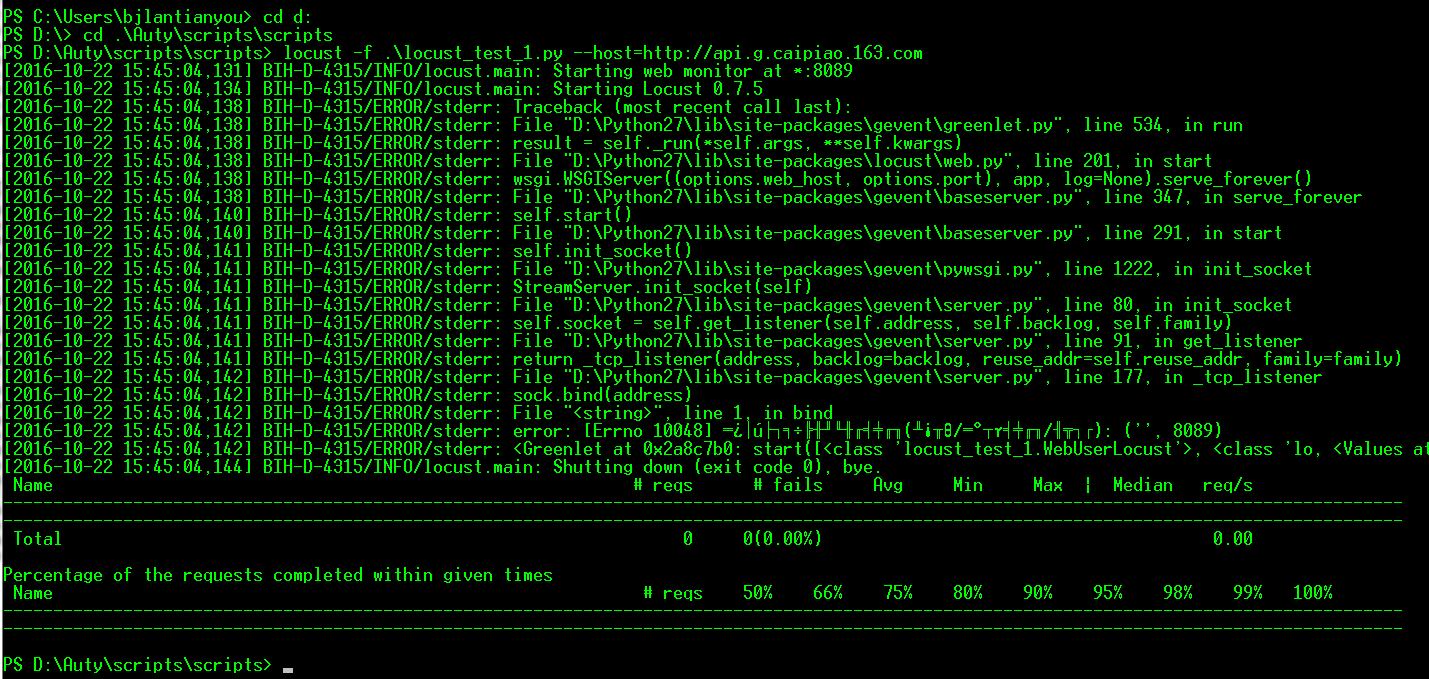function checkPid($result,$port){
$port =$port.split(":")
if(($result.split()).split(":")[($result.split()).split(":").Count-1] -eq $port){$tPid = ($result.split())[($result.split()).count-1]
if($tPid -ne "0"){ Write-Host "您查询的端口被以下程序占用：" -ForegroundColor Red$target = tasklist|findstr $tPid Write-Host$target
$sig =$true
}else{
$sig =$false
}
}else{
$sig =$false
}
$sig } function checkPort($port){
$port = ":" +$port
$results = netstat -ano|findstr$port
if($results.count -gt 0){ if($results.count -eq 1){
$sig = checkPid$results $port if($sig -eq $false){ Write-Host "您所查询的端口未被占用！" -ForegroundColor Green } }else{ foreach($result in $results){ if($result){
$sig = checkPid$result $port if($sig -eq $true){ break } } } if($sig -eq $false){ Write-Host "您所查询的端口未被占用！" -ForegroundColor Green } } }else{ Write-Host "您所查询的端口未被占用！" -ForegroundColor Green } }$port = $null while($port -ne "exit()"){
$port = Read-Host "请输入要查询的端口号" if($port -eq "exit()"){
break
}
checkPort \$port
}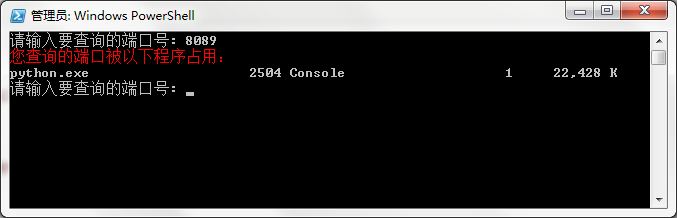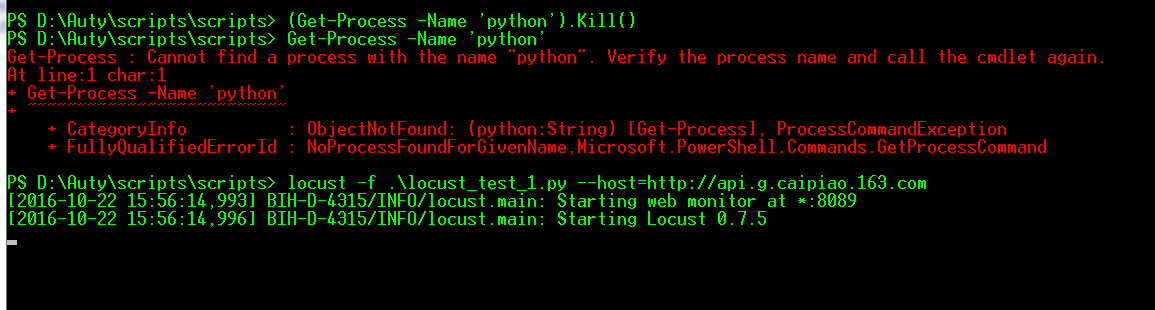locust -f .\locust_test_1.py --host='http://api.winyyg.com' --no-web -c 1000 -r 10 -n 1000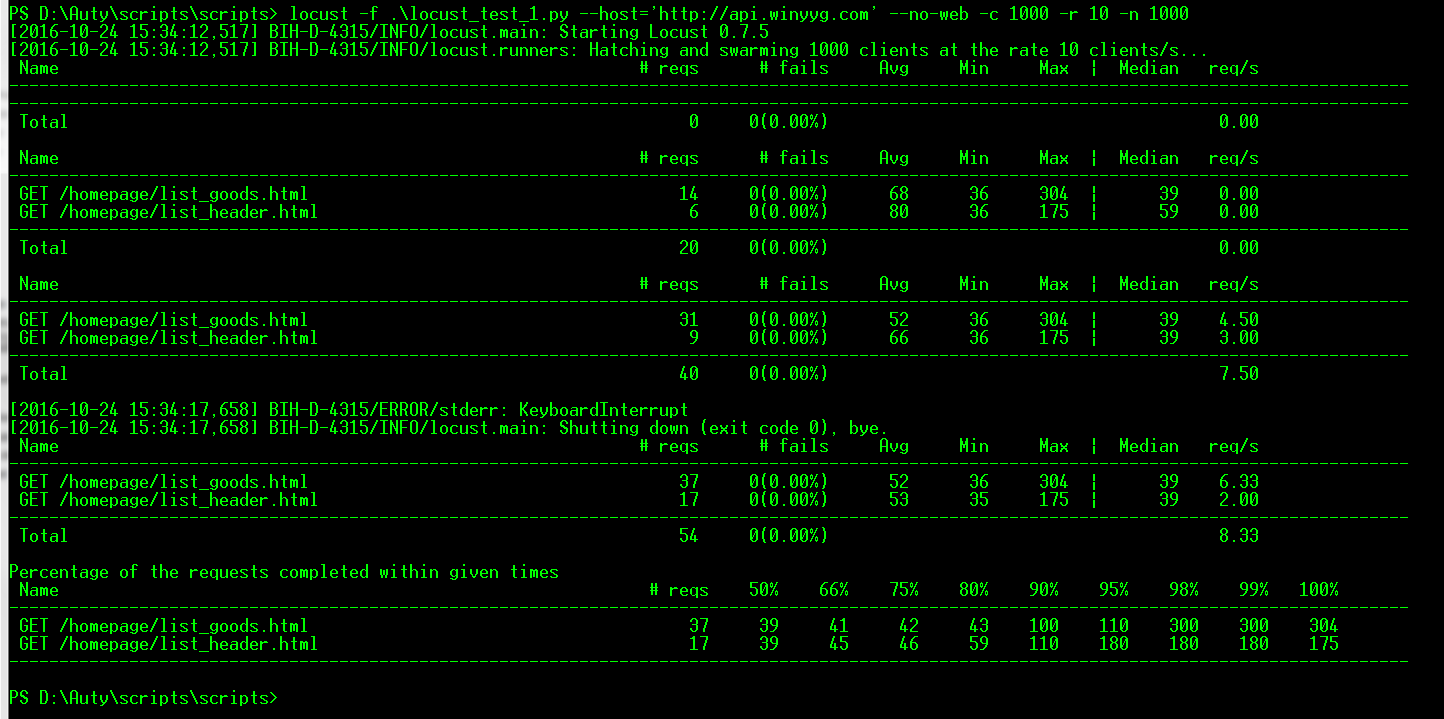## Locust负载测试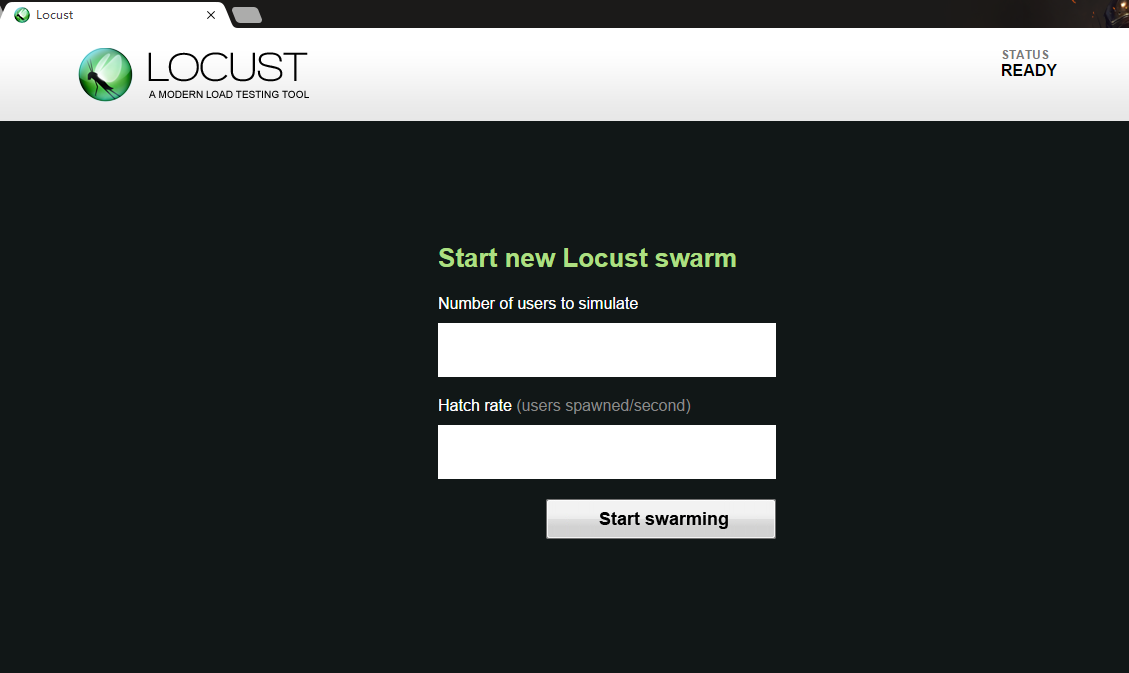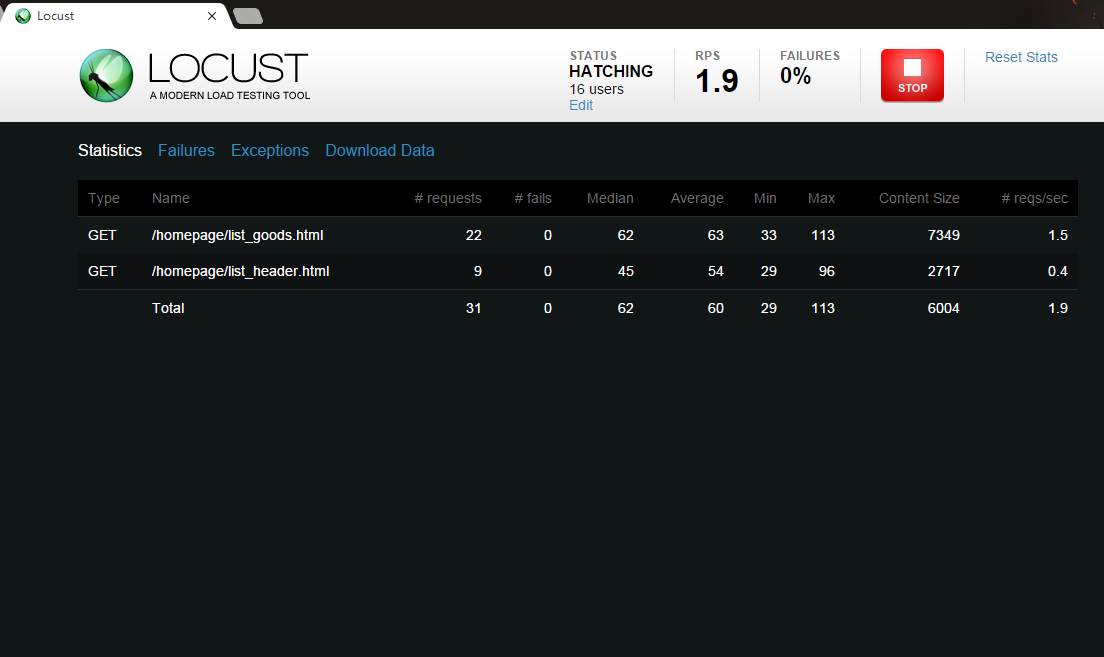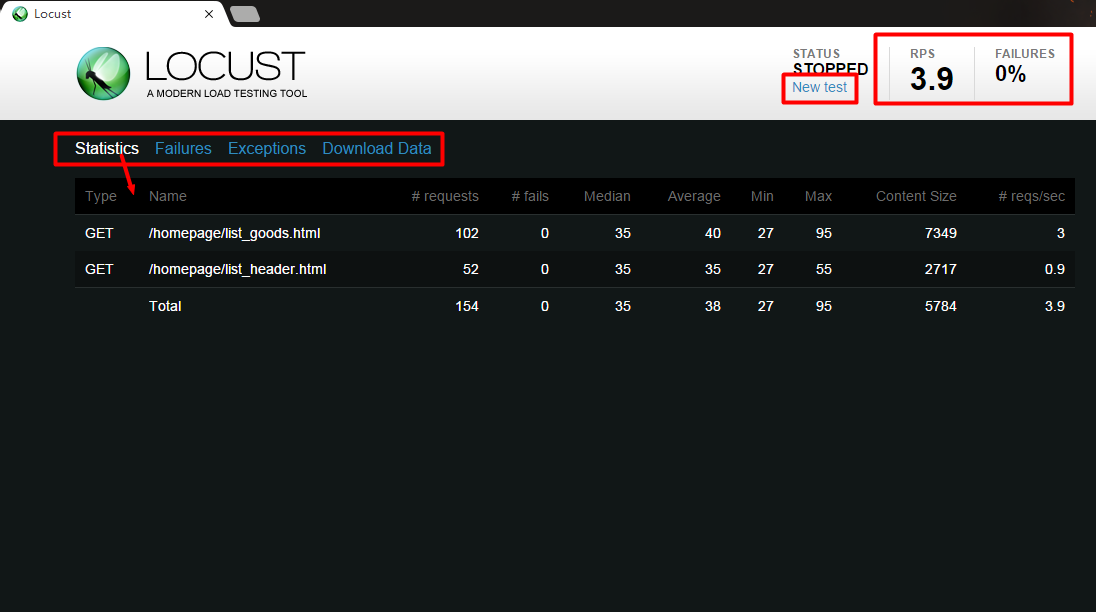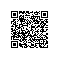使用钉钉扫一扫加入圈子
+ 订阅